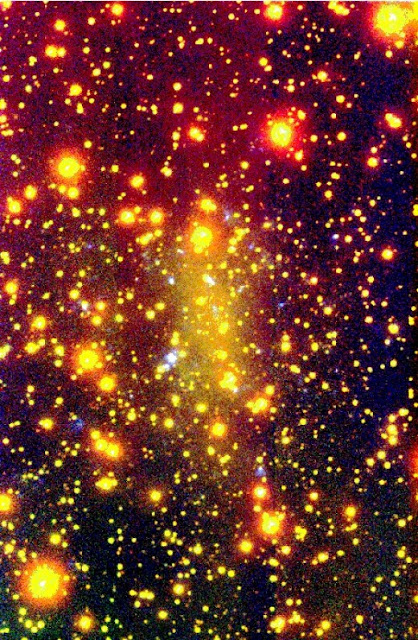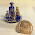### An Existential Definition of the Binary Encoding of the Universe

Latest update: July 25, 2022. Page URL indicates original publication date; meanwhile, times change and the updates continue.

## Theory of a Physical Reality Philosophy -A Math - Physics - Metaphysics Approach

#### Alternate titles

• How to Interpret 0 and 1
• The Numbers 0 and 1 Defines Nonexistence versus Existence
• What Is the Mathematical and Metaphysical Concept and Significance of the Numbers 0 and 1
• 1 - The First Non Zero Number - What Is the Meaning of Zero and One
• Binary Reality Fact of Existence
• It's All Ones and Zeros
• Does Negative Zero ExistOne Represented by Many

## The Basic Interpretation of 0 and 1

This is a somewhat metaphysical approach as to the existence, meaning, concept, and significance of the numbers 0 and 1. What are the meanings of 0 and 1? What does 0 and 1 really signify?

Zero and One are the most important numbers of all the numbers in the universe. Zero and One defines the difference between existence (1) and nonexistence (0). All other numbers signifying existence can only exist when 1 exists. One is the opposite of none.

1 and 0 are the demarcation defining what is and what is not.

All numbers other than 1 are more of 1 or are none.

0 and 1 are the only numbers times themselves that are themselves. All other numbers become other numbers.

A successful division of 1 by a number other than 1 can only occur when the 1 is not a true 1. The 1 was, in fact, the sum of smaller 1's.

1 is not the first prime number. Contrary to popular belief, 1 is not a prime number at all.

0 exists when 1 or 1's do not exist. -1 cannot exist unless 1 exists. -0 does not exist. or does it?

## Does -0 Exist? Otherwise known as Does the Concept of Negative Zero Exist?

If -0 equals 1, then -1 would equal 0; but it does not. So in math, -0 does not exist.

Logic and metaphysical logic, however could be different. Negative can mean not or can mean opposite. A Not 1 means it is equal to anything other than one. An opposite to one would be equal to 0. So not 1 and opposite to 1 have different meanings.

Applying the same logic to zero, however, gives us different results. A not zero means it is equal to anything other than zero; an opposite to zero means it is equal to anything other than zero. So not zero and opposite to zero are equal, whereas not one and opposite to one are not.

It therefore follows that stating -0 is also stating that the concept of not is the same as the concept of opposite. In other words, not equals opposite. That is not true. The meaning of not does not mean the same as the meaning of opposite. So -0 must be interpreted as a false statement, thus -0 does indeed not exist.

## The Diverse or Abstract Interpretation of 1 and the Universe

The concept of the number 1 is greatly dependent on the undefined premise of "one what?". Every time science thinks it has found a true one, it invariably turns out to not be so. As an example, the atom was once thought to be the one true basic building block of the universe. But then it turned out the atoms were composed of the smaller 1's of electrons, protons, and neutrons. And now we have quarks, leptons, strangelets, etc. to contend with.

Who knows? Maybe there is no true one. Everything will always be discovered to be composed of something smaller. However, in its most basic form (if we can ever find it), all else derives from one. One is the definition, reality, concept, and source of all existence.

### The Binary Base 2 Numbering System

Nothing versus something is the root of the binary system of numbers.

We use Base 10 in our day-to-day living. Base 10 has ten numbers (0-9) and orders of magnitude that are times ten. The lowest-order number represents itself times one. The next-order number represents itself times ten. The next order number represents itself times 10x10 or itself times 100. And so on.

An example would be the number 742. This number means that there are:
• two 1’s,
• four 10’s,
• and seven 100’s.
Which represents 2 + 40 + 700; for a total of 742.

The base 2 binary number system uses the same structure, the only difference being the order of magnitude. Base 2 has two numbers (0 and 1) and orders of magnitude that are times two. The lowest-order number represents itself times one. The next-order number represents itself times 2. The next order number represents itself times 2x2 or itself times 4. And so on.

An example would be the number 110. This number means that there are:
• No 1’s,
• one 2,
• and one 4.
Which represents 0 + 2 + 4; for a total of 6.

Other binary examples are:
• 0=0, 1=1
• 10=2, 11=3
• 100=4, 101=5, 110=6, 111=7
• 1000=8, 1001=9, 1010=10, 1011=11, 1100=12, 1101=13, 1110=14, 1111=15
• 10000=16

Binary is the mathematical representation of how the universe encodes itself.

Here are the quick lessons on this and the other: Base Number Counting Systems. All on one page.
.

### 1 and the Base 10 Pyramid

And what page about the base 10 number 1 would be complete without the usual acknowledgement of the series of 1's times themselves (squared)...

• 1² = 1
• 11² = 121
• 111² = 12321
• 1111² = 1234321
• 11111² = 123454321
• 111111² = 12345654321
• 1111111² = 1234567654321
• 11111111² = 123456787654321
• 111111111² = 12345678987654321

#### The binary paradigm is the foundation of the universe, including fractals (recursive geometric shapes) and chaos theory (the butterfly effect).NASA deep space photo demonstrating fractals/chaos theory

#### There is no reason to believe the concept of "many" excludes the concept of multiple realities.A visual representation of the theory of parallel and/or alternate universes.

#### Humankind relative to the universe

There are no extra pieces in the universe (-Deepak Chopra). You are here for a reason.

- End of Article -

Re: Using Mobile?
Home: site intro and featured articles/resources.
View Web Version: displays Main Menu article categories (will be located below), additional site info (below and side), search function, translation function.

1.Interesting article.
i have been thinking about this and i cannot find any actual example of zero in the real universe. (I do not count "absence of something" to be "something.") So, I believe zero only exists as a concept of the mind. in fact, i am close to deciding that all numbers are really just useful concepts.

we use math very well to describe and explain the physical world. conceptual models are great for working with the actual world. but we should not take that to mean that these concepts are ultimate truth, but merely close enough for our purposes.

Quantum physics comes closer to revealing reality, but even that model falls short of explaining the real universe.

2.The field of zero energy is space,0.
0,zero is not nothing,it’s the fulcrum for positive and negative energy.+0-.plus and minus cancel each other out to make nothing or 0 or empty space.no field can have balance if it does not contain a opposite.
So zero energy or space must be made of a equal amount of positive and negative energy that cancels each other out to create the balance of a empty zero state energy field called space.if we break down the universe to its smallest part the atom,we see that it’s made with the neutron,the positive proton and the negative electron.if we marry the proton and electron together they will nullify each other out creating empty space.
The first atom was created by a small spark that was caused by static.the coldness of space would effect the field as we see with super conductors.
A black hole shows how the huge electromagnetic gravitation effects the atoms of matter,it stripes the electrons and protons apart from matter and simply joins the positive and negative energy together to form space,a field of zero energy but with the most potential energy.
If anyone is interested I can explain the nature of counter space displacement due to matter occupying the empty space where counter space comes from.
For any matter to exist it has to share its position with counter space,we feel the effects of counter space as gravity.
Many thanks leyton glover

Alas. Comments containing spam, profanity, etc. are deleted.